# Gravitation

#### Acceleration due to gravity

a) Acceleration produced in a body due to the force of gravity is termed as acceleration due to gravity.
b) The acceleration due to gravity is the rate of increase of velocity of a body falling towards the earth.
c) The acceleration due to gravity is equal to the force by which earth attracts a body of unit mass towards its centre.
d) Let 'm' be the mass of body and 'F' be the force of attraction at a distance 'r' from the centre of earth, then acceleration due to gravity (g) at that place will be g =, where Me = mass of earth.
e) The expression g =is free from 'm' (mass of body). This means that the value of 'g' does not dependupon the shape, size and mass of the body. Hence if two bodies of different masses, shapes and sizes are allowed to fall freely, they will have the same acceleration. If they are allowed to fall form the same height,they will reach the earth simultaneously.
f) The acceleration of a body on the surface of the earth is g = 9.80 m/s2 or 981 cm/s2.
g) Dimensional formula of g is [M0 L1 T-2].
h) The value of acceleration due to gravity depends on the following factors.
Height above the surface of earth :
(i) As we go above the surface of the earth, the value of 'g' decreases. Consider a point P at a distance r form the centre of earth.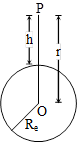The acceleration due to gravity at point P is.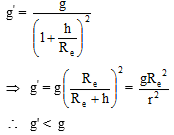(ii) As we go above the surface of the earth, the value of 'g' decreases. g' ∝for r > Re
(iii) If h < < Re, (according to binomial expansion) g'= g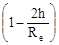(iv) If r = ∞ , g'= 0. At infinite distance form the earth, the value of 'g' becomes zero.
(v) Value of g at the surface of earth (h = 0), i.e.(b) Below the surface of earth :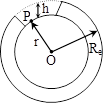(i) The value of 'g' decreases on going below the surface of the earth.
(ii) The value of 'g' at a distance h below this earth's surface be gh and 'g' at the earth's surface then -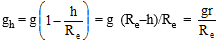i.e gh < g, r is the distance form the centre of the earth (r< Re), r = Re - h.
(iii) If d is the density of the earth then the force on pt. P is(iv) At the centre of the earth, h = Re (i.e r = 0) so g = 0.
(v) Value of 'g' is maximum at the surface of earth.
(vi) Graphical representation of variation in the value of g
Variation of g with distance r from the centre of earth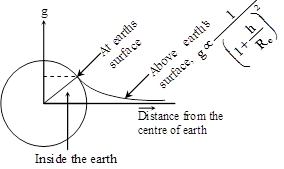(c)Variation in value of 'g' on the surface of earth :
It is due to two reasons :
(1) Due to shape of earth :
(i) The earth is elliptical in shape. It is flattened at the poles and bulged out at the equator. Now, we know that g µ 1/Re2, therefore the value of g at the equator is minimum and the value of g at the poles is maximum (Q Radius at poles is < Radius at equator,)⇒ Re > Rp, Hence, ge < gp
(ii)(iii) Numerical value of Rp is twenty one kilometre less than Re. Therefore gp - ge= 0.02m/s2.
(2)Due to rotation of earth :(i) Earth is rotating about its own axis form west to earth with an angular velocity w.
(ii) On a latitude λ, point P is moving in circle with radius 'r'. If we keep a body then some part of its gravitational force will be used up for providing centripetal force, therefore there is reduction in total gravitational force. As a result of this, value of 'g' decreases.
(iii) If w is the angular velocity of rotation of the earth, Re is radius of the earth, then the observed value of g at the latitude is represented by g' then.
g' = g0 - ω2 Re cos2 λ
or g' = g0 - 0.0337cos2 λ
where g0 is value of 'g' at the poles.
(iv) At equator, λ = 0 º
g' = g0- ω2 Re (Minimum value)
= g - 0.0337
(v) At poles, λ = 90º, cos = 0 and g' = g (Maximum value)
(vi) From the above expressions we can conclude that the value of 'g' at the surface of earth is maximum at poles and minimum at the equator. Therefore weight of bodies is maximum at the poles and will go ondecreasing towards the equator. (it is minimum at the equator).
(vii) If earth stops rotating about its axis (ω = 0), the value of g will increase everywhere, except at the poles. On the contrary, if there is increase in the angular velocity of earth, then except at the poles the value of 'g' will decrease atall places.
(viii) Maximum effect of rotation takes place at the equator while at poles, there is no effect.
(ix) If ω =then, at equator weight of body will become zero but its mass remains unaltered.
a) That means if the earth starts rotating with an angular speed 17 times the present,
b) If g equator = 0, in this condition , time period of earth's rotation will become 1.41 hours instead of 24 hours.

If you want to give information about online courses to other students, then share it with more and more on Facebook, Twitter, Google Plus. The more the shares will be, the more students will benefit. The share buttons are given below for your convenience.

#### IIT (Class X)

• Unit, Dimension & Error
• Vectors
• Motion in One Dimension
• PROJECTILE MOTION
• NEWTON'S LAWS OF MOTION & FRICTION
• WORK, POWER, ENERGY & CONSERVATION LAWS
• CIRCULAR MOTION & ROTATIONAL DYNAMICS
• GRAVITATION